Email Us: info@lupinepublishers.comCall Us: +1 (914) 407-6109   57 West 57th Street, 3rd floor, New York - NY 10019, USA

# Lupine Publishers Group

## Lupine Publishers

Submit Manuscript

ISSN: 2637-4579# Optical and Geometrical Characteristics of Secondary Conic Concentrators Volume 3 - Issue 4

Akbarov R Yu and Nurmatov Sh R*

• Institute of Materials Science of the Uzbek Academy of Sciences, Uzbekistan

Received: October 01, 2019;   Published: October 10, 2019

*Corresponding author: Nurmatov Sh R, Institute of Materials Science of the Uzbek Academy of Sciences, Tashkent city, Yunusabad district, Chingiz Aitmatov St., Building 2B, Uzbekistan

DOI: 10.32474/OAJBEB.2019.03.000169

## Abstract

In this work the task of increasing the efficiency of solar collectors using secondary conical concentrators is considered. The article presents the analytical and numerical analysis of the optical-geometrical characteristics of the secondary conic concentrators.

Keywords: Solar concentrator; collector; secondary concentrator; radiation; flux density; focon; parabolic; conical; reflector; parametric angle; critical angle; length; radius; large solar furnace

## Introduction

In solar engineering, secondary concentrators are commonly used to increase the density of concentrated energy flux generated from the primary solar concentrators. They can also be used, for example, to change the location of the focal area of the concentrator or the shape of the distribution of concentrated flux density, etc. Note that the modules of secondary concentrators can be used without a primary concentrator, but with a lower concentration ratio.

The types of secondary concentrators are very different, typically they are parabolic concentrators, various types of focons (parabolotoric, conical, wedge-shaped, prism etc.), lenses, parabolic through concentrators, flat reflectors, fragments of conical sections - cassegrain system, gregoria system etc.

For certain categories of concentrators used as secondary concentrators, their optical-geometric properties have been studied in enough detail.

The achieved positive effect using secondary concentrators depends on the particular optical scheme, which includes the secondary concentrator. Therefore, despite the well-known general optical-geometrical properties of several types of concentrators, for the “primary concentrator + secondary concentrator” optical system, a detailed analysis of the output characteristics of such systems is difficult or evaluative. Therefore, in these cases a detailed optical- geometrical calculation of specific system is separately performed based on the developed mathematical models [1,2].

This paper investigates the optical and geometrical properties of conical concentrators.

We introduce the notations as shown in Figure 1. The geometry of the conical focon is defined by two radii (R and r), angles γ (parametric angle) and θ (critical angle), and length L, and three parameters are needed to uniquely determine the focon geometry. We have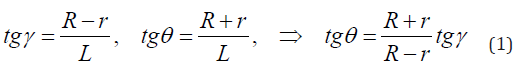And for these angles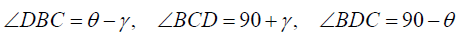Let φ will be the angle of incidence and reflection at point D (the vector DN is the normal to the surface), and β the angle between the reflected beam 1 and the direct CD. Then it is easy to see the correctness of the following relations: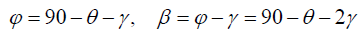Let us determine such parameters of the focon, for which all the rays with an angle of incidence greater than θ come back. A necessary condition for this case is the equality β = 0 at the point D (that is, the reflected beam begins go to back). For this case we have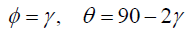It’s obvious that, in this case, the focon geometry is determined by two parameters for the given θ.

We have(It is easy to see that for the case of multiple reflection i, we have γ = (90-θ/ 2i).

For such conditions, the angles cannot be arbitrary. Figure 2 presents a graph in which a possible interval of angles is highlighted. Thus, the values of the angle γ must be less than 30 degrees.

Now we define the output radius r and the length L of the focon. From equation (1) we have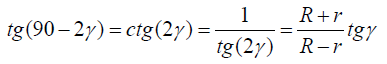orfrom here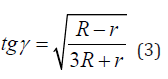And, in turn,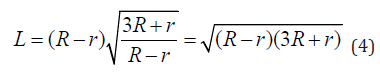From equation (1) we define r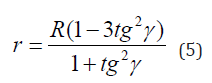Since r>0, therefore 1-3tg2γ > 0 or tgγ <, and hence we have γ <300, i.e. as mentioned above.

Thus, it can be noted that the value of the critical focal angle determines such a focal configuration (θ = 90 − 2γ ) that rays with an incidence angle greater than θ always return back. In this case, if the angle of incidence of the rays is less than θ, then after a single or multiple reflection, they can pass through the focon or come back.

Based on the obtained relations the calculations were carried out to determine the parameters of the conical focon in the case of its use in the optical scheme of the thousand kWt Large Solar Furnace of the Institute of Materials Science (Uzbekistan, LSF) [3,4]. In Figure 3 the dependence of the output radius and the length of the focon on its parametric angle is shown. Based on these data, it is possible to determine the optimal geometry of the conical focon for the LSF.

1. R Winston, JC Miсano, P Benitez (2005) “Nonimaging Optics”, Elsevier- Academic Press, New York, USA.
2. Zakhidov RA, Weiner AA, Umarov G (1992) Theory and Calculation of Applied Solar Energy Concentrating Systems (English Ed). Gujarat Energy Development Agency, Vadodara, India, pp. 146.
3. Akbarov R Yu, Paizullakhanov MS (2018) Characteristic Features of the Energy Modes of a Large Solar Furnace with a Capacity of 1000 kW. Applied Solar Energy 54(2): 99-109.
4. Akbarov R (2019) Thousand kW High-Temperature Solar Furnace in Parkent (Uzbekistan) – Energetical Characteristics.
Close## Online Submission System

### Submit ManuscriptClick here for Manuscript Sample Templates### Drag and drop files here

or

( For multiple files submission, zip them in a single file to submit. For file zipping software Download )